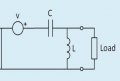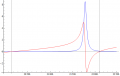# resonance in rlc circuits

#### joshpig

Joined Jan 26, 2016
4
Hi!
If you want to find the resonant frequency for the voltage and current in a load (load is a combination of resistor, capacitor, inductor), in a circuit which has another capacitor and inductor. Would you use just the components outside the load, or include those in the load in the equation 1/(2pi)(LC^1/2).?

I'm not sure if I've explained this very well but we shall see)Thanks

Last edited:

#### Dodgydave

Joined Jun 22, 2012
10,681
The resonant frequency is when Xc = Xl,

•joshpig

#### ErnieM

Joined Apr 24, 2011
8,353
You need to work out an equivalent circuit where there is but a single resistor, capacitor, and inductor.

Then Dave's doggy formula works perfectly.

•joshpig

#### The Electrician

Joined Oct 9, 2007
2,916
Hi!
If you want to find the resonant frequency for the voltage and current in a load (load is a combination of resistor, capacitor, inductor), in a circuit which has another capacitor and inductor. Would you use just the components outside the load, or include those in the load in the equation 1/(2pi)(LC^1/2).?

I'm not sure if I've explained this very well but we shall see)
View attachment 99452
Thanks
It all depends on your definition of resonance. For example, see this page for the three common definitions of resonance: http://hyperphysics.phy-astr.gsu.edu/hbase/electric/parres.html

Your circuit is not exactly like that one, but the given definitions of resonance will give different results when applied to your problem.

Which definition of resonance will you use?

•joshpig

#### joshpig

Joined Jan 26, 2016
4
Thank you for the fast responsesI am just trying to find the frequency at which current flowing through the load and the voltage across it to be in phase, now i was guessing that this is the resonant frequency as that is when the circuit is purely resistive? is that correct?

And is Xi/Xc referring to the impedance ?

#### Dodgydave

Joined Jun 22, 2012
10,681
Xl and Xc are the reactances(AC resistance) of the inductor and capacitor,your circuit has a v+ symbol, is it a dc supply?

•joshpig

#### The Electrician

Joined Oct 9, 2007
2,916
Thank you for the fast responsesI am just trying to find the frequency at which current flowing through the load and the voltage across it to be in phase, now i was guessing that this is the resonant frequency as that is when the circuit is purely resistive? is that correct?

And is Xi/Xc referring to the impedance ?
You said that your "load is a combination of resistor, capacitor, inductor". Show the circuit of the load in detail. Together with the additional L and C you showed in post #1, the expression for the frequency at which the load current is in phase with the load voltage can be determined.

•joshpig

#### joshpig

Joined Jan 26, 2016
4

#### Brownout

Joined Jan 10, 2012
2,390
You have to consider all reactances. However, you can simplify things a little by considering only the circuit to the left (initially) and making a thevinen equivalent.

•joshpig

#### The Electrician

Joined Oct 9, 2007
2,916
Last edited:
•joshpig

#### joshpig

Joined Jan 26, 2016
4
Wow brilliant thanks! I would love to see how you did that, if you have that derivation to hand it would be great to see it?

#### The Electrician

Joined Oct 9, 2007
2,916

#### The Electrician

Joined Oct 9, 2007
2,916
To get some more insight into the behavior around resonance, let's plot both the real part (blue) and the imaginary part (red) of the impedance:The real part becomes very small at the lowest and highest resonance frequencies. At the middle resonance, ω = 21778.5, the real part is 8.46 ohms, a more reasonable value. The resonance there is the useful one. It's a fairly high Q resonance but the external inductance was treated as ideal.

Here's the calculation of the value of the real part at the 3 resonances:•joshpig